Access to comprehensive ICSE S Chand Maths Class 9 Solutions Chapter 19 Trigonometrical Ratios Chapter Test encourages independent learning.

## S Chand Class 9 ICSE Maths Solutions Chapter 19 Trigonometrical Ratios Chapter Test

Question 1.
If A = 30° and B = 60°, then which of the following is/are correct?
I. sin A + sin B = cos A + cos B
II. tan A + tan B = cot A + cot B
Select the correct answer using the code given below:
(a) Only I
(b) Only II
(c) Both I and II
(d) Neither I nor II
Solution:
A = 30° and B = 60°
I. sin A + sin B = cos A + cos B
L.H.S. = sin A + sin B = sin 30° + sin 60°
= $$\frac { 1 }{ 2 }$$ + $$\frac{\sqrt{3}}{2}$$ = $$\frac{1+\sqrt{3}}{2}$$
R.H.S. = cos 30° + cos 60°
= $$\frac{\sqrt{3}}{2}$$ + $$\frac { 1 }{ 2 }$$ = $$\frac{1+\sqrt{3}}{2}$$
∴ L.H.S. = R.H.S.

II. L.H.S. tan A + tan B = tan 30° + tan 60°
= $$\frac{1}{\sqrt{3}}$$ + √3 = $$\frac{1+3}{\sqrt{3}}$$ = $$\frac{4}{\sqrt{3}}$$
R.H.S. = cot A + cot B = cot 30° + cot 60°
= √3 + $$\frac{1}{\sqrt{3}}$$ = $$\frac{3+1}{\sqrt{3}}$$ = $$\frac{4}{\sqrt{3}}$$
∴ L.H.S. = R.H.S.
∴ Both I and II are correct. (c)Question 2.
What is cot 15° cot 20° cot 70° cot 75° equal to?
(a) -1
(b) 0
(c) 1
(d) 2
Solution:
cot 15° cot 20° cot 70° cot 75° = cot (90° – 75°) cot (90° -70°) cot 70° cot 75°
= tan 75° tan 70° cot 70° cot 75°
= tan 75° × cot 75° tan 70° × cot 70° (∵ tan θ cot θ = 1)
= 1 × 1 = 1

Question 3.
(i) If sin 3 A = cos ( A – 2°) where 3 A and
(A – 2°) are acute angles, what is the value of A?
(a) 22°
(b) 23°
(c) 24°
(d) 25°
(ii) If sin (x – 2y) = cos (4y – x), then the value of cot 2y is:
(a) 0
(b) 1
(c) $$\frac{1}{\sqrt{3}}$$
(d) undefined
Solution:
(i) sin 3 A = cos ( A – 2°), 3 A and ( A – 2°) are acute angles
sin 3 A = cos (A – 2°) = sin {90° – A + 2°}
Comparing both sides,
3A = 90° – A + 2° ⇒ 3A + A = 92°
⇒ 4A = 92° ⇒ A = $$\frac{92^{\circ}}{4}$$ = 23°
∴ A = 23°

(ii) sin (x – 2y) = cos (4y – x)
sin (x – 2y) = sin (90° – 4y + x)
Comparing,
x – 2y = 90° – 4y + x
4y – 2y = 90° ⇒ 2y = 90° ⇒ y = 45°
Now, cot 2y = cot (2 × 45°) = cot 90°
not defined (d)

Question 4.
(i) What is the value of cos 1° cos 2° cos 3° ………… cos 90°?
(a) $$\frac { 1 }{ 2 }$$
(b) 0
(c) 1
(d) 2

(ii) If tan $$\left(90^{\circ}-\frac{\mathrm{A}}{2}\right)=\sqrt{3}$$, then value of A is:
(a) 0
(b) $$\frac{1}{\sqrt{2}}$$
(c) $$\frac { 1 }{ 2 }$$
(d) 1
Solution:
(i) cos 1° cos 2° cos 3° ………. cos 90°
∵ cos 90° = 0
∴ cos 1° cos 2° cos 3° …….. cos 90° = 0

(ii) tan $$\left(90^{\circ}-\frac{A}{2}\right)=\sqrt{3}$$ = tan 60°
Comparing, we get
90° – $$\frac { A }{ 2 }$$ = 60° ⇒ $$\frac { A }{ 2 }$$ = 90° – 60° = 30°
∴ A = 30° × 2 = 60°
Now cos A = cos 60° = $$\frac { 1 }{ 2 }$$ (c)

Question 5.
(i) In a △ABC, ∠ABC = 90°, ∠ACB = 30°, AB = 5 cm. What is the length of AC?
(a) 10 cm
(b) 5 cm
(c) 5 √2 cm
(d) 5 √3 cm
(ii) If ABC is a right-angled triangle at C having u units, v units and w units as the lengths of its sides opposite to the vertices A, B, C respectively, then what is tan A + tan B equal to?Solution:
In △ABC, ∠ABC = 90°, ∠ACB = 30°, AB = 5 cm
(i) sin 30° = $$\frac{\text { AB }}{\text { AC }}$$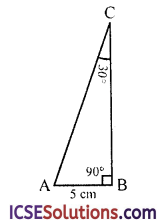⇒ $$\frac { 1 }{ 2 }$$ = $$\frac{5}{\text { AC }}$$
⇒ AC = 2 × 5 = 10 cm

(ii) tan A + tan B= $$\frac { u }{ v }$$ + $$\frac { v }{ u }$$ = $$\frac{u^2+v^2}{u v}$$
But w2 = u2 + v2 (Pythagoras Theorem)
∴ $$\frac{w^2}{u v}$$ (d)

Question 6.
(i) If cot A =$$\frac { 8 }{ 15 }$$, then what is the value of $$\sqrt{\frac{1-\cos A}{1+\cos A}}$$ where A is a positive acute angle.
(a) $$\frac { 1 }{ 5 }$$
(b) $$\frac { 2 }{ 5 }$$
(c) $$\frac { 3 }{ 5 }$$
(d) $$\frac { 4 }{ 5 }$$

(ii) If A is an acute angle and sin A = $$\sqrt{\frac{x-1}{2 x}},$$ then what is tan A equal to?Solution:
(i) cot A = $$\frac { 8 }{ 15 }$$
∴ In right △ABC
cot A = $$\frac{\text { AC }}{\text { BC }}$$ = $$\frac { 8 }{ 15 }$$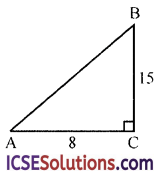∴AC = 8, BC = 15
Then AB2 = AC2 + BC2 = 82 + 152
= 64 + 225 = 289 = (17)2
∴ AB = 17
cos A = $$\frac{\text { AC }}{\text { AB }}$$ = $$\frac { 8 }{ 17 }$$(ii) sin A = $$\sqrt{\frac{x-1}{2 x}}$$ = $$\frac{\mathrm{BC}}{\mathrm{AB}}$$ = $$\frac{\sqrt{x-1}}{\sqrt{2 x}}$$
In the △ABC,
BC = $$\sqrt{x-1}$$, AB = $$\sqrt{2 x}$$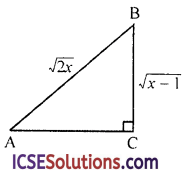∴ AC2 = AB2 – BC2 = $$(\sqrt{2 x})^2$$ – $$(\sqrt{x-1})^2$$
= 2x – (x – 1) = 2x – x + 1
= x + 1
∴ AC = $$\sqrt{x+1}$$
Now, tan A = $$\frac{\text { BC }}{\text { AB }}$$ = $$\frac{\sqrt{x-1}}{\sqrt{x+1}}$$
= $$\frac{\sqrt{x-1}}{\sqrt{x+1}}$$Question 7.
What is the value of sin3 60° cot 30° – 2 sec2 45° + 3 cos 60° tan 45° – tan° 60°?
(a) $$\frac { 35 }{ 8 }$$
(b) $$\frac { -35 }{ 8 }$$
(c) $$\frac { -11 }{ 8 }$$
(d) $$\frac { 11 }{ 8 }$$
Solution:
sin3 60° cot 30° – 2 sec2 45° + 3 cos 60° tan 45° – tan2 60°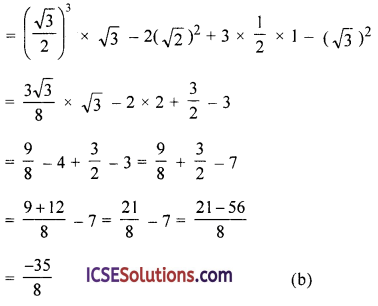Question 8.
(i) If x + y = 90° and sin x : sin y = √3 : 1 then what is x : y equal to
(a) 1 : 1
(b) 1 : 2
(c) 2 : 1
(d) 3 : 2

(ii) If sin x = cos y and angle x and angle y are acute, then what is the relation between x and y?
(a) x = y = $$\frac{\pi}{2}$$
(b) x + y = $$\frac{3\pi}{2}$$
(c) x + y = $$\frac{\pi}{2}$$
(d) x + y = $$\frac{\pi}{4}$$
Solution:
(i) x + y = 90°, $$\frac{\sin x}{\sin y}$$ = $$\frac{\sqrt{3}}{1}$$
y = 90 – x°
$$\frac{\sin x}{\sin \left(90^{\circ}-x\right)}$$ = $$\frac{\sqrt{3}}{1}$$
⇒ $$\frac{\sin x}{\cos x}$$ = $$\frac{\sqrt{3}}{1}$$ tan x = √3
⇒ tan x = tam 60°
∴ x = 60°
and y = 90° – 60° = 30°
∴ x : y = 60° : 30° ⇒ x : y = 2 : 1 (c)

(ii) sin x = cos y = x and y are acute angle
sin x = cos y = sin (90° – y) {∵ cos θ = sin (90° – θ)}
∴ x = 90° – y x + y = 90° = $$\frac{\pi}{2}$$

Question 9.
(i) What is the value of the expression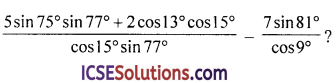(a) -1
(b) 0
(c) 1
(d) 2

(ii) What is the value of the expression(a) 8
(b) 10
(c) 16
(d) 18
Solution: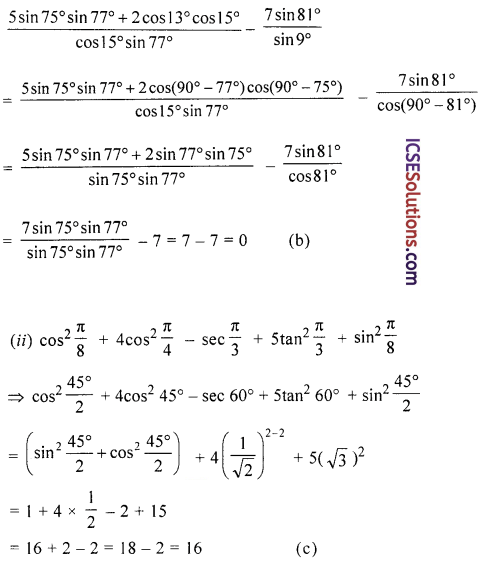Question 10.
In the figure (not drawn to scale) a rocket is fired vertically upwards from its launching pad P. It first rises 20 km vertically up and then travels 80 km at 30° to the vertical. PA respresents the first state of its journey and AB the second; C is point vertically below B on the same horizontal level as P. Calculate:
(i) the height of the rocket when it is at point B.
(ii) the horizontal distance of point C from point P.Solution:
In the given figure,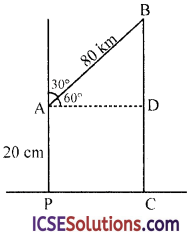A rockets is launched from P 20 km to A then 80 km to B making an angle of 30° with PA.
We have to find PC from A, draw AD || PC, Then AD = PC
Then ∠BAD = 90° – 30° = 60°
cos θ = $$\frac{\text { AD }}{\text { AB }}$$ ⇒ cos 60° = $$\frac{\text { AD }}{\text { AB }}$$
⇒ $$\frac { 1 }{ 2 }$$ = $$\frac{\mathrm{PC}}{80}$$ = PC = $$\frac { 80 }{ 2 }$$ = 40 Km
and sin 60° = $$\frac{\text { BD }}{\text { AB }}$$ ⇒ $$\frac{\sqrt{3}}{2}$$ = $$\frac{\mathrm{BD}}{80}$$
⇒ BD = $$\frac{80 \sqrt{3}}{2}$$ = 40√3 Km
∴ BC = BD + DC = BD + AP
= (40√3 + 20) Km